# Organic Chemistry : Finding Oxidation Number

## Example Questions

### Example Question #1 : Finding Oxidation Number

The oxidation numbers of calcium and chromium in the compound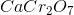are __________ and __________, respectively.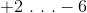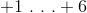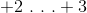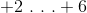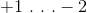Explanation:

Calcium is in the second group of the periodic table, so its oxidation number will always be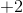. This is true for all alkaline earth metals.

As for chromium, the dichromate anion (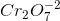) has an overall charge of. Each oxygen atom will always have an oxidation number ofas well, so the oxygens would have a total negative charge of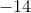. The sum of the oxidation numbers have to equal the overall charge, so chromium must be used to balance the negative charges from the oxygen. To bring the overall charge to, the two chromiums have to have total charge of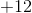, giving each an oxidation number of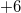.

### Example Question #1 : Oxidation Reduction Reactions

What is the oxidation number of the manganese in potassium permanganate?None of these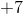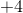Explanation:

Manganese is a transition metal, so its oxidation number is more variable than the other elements of the compound. Oxygen is almost always. Potassium is almost always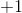. The sum of the oxygens and the potassium is.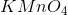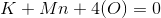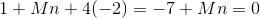The compound is neutral, so the manganese isto balance the net molecular charge.

### Example Question #1 : Finding Oxidation Number

Compared to oxygen in water, the oxygen in hydrogen peroxide has __________ valence electron(s).

One more

The same

Two more

One less

One less

Explanation:

To solve this question we need to calculate the oxidation number of oxygen in both molecules. The formula for water is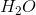. The oxidation number of hydrogen is +1. Since there are two of them, the hydrogen atoms contribute to a charge of +2. The water molecule is neutral; therefore, the oxygen must have an oxidation number ofto balance the charge. The formula for hydrogen peroxide is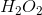. Using the same logic as water, we can determine that hydrogen contributes +2. We have two oxygen atoms in this case; therefore, each oxygen atom will have an oxidation number ofto give a charge of. This will balance the charges and provide a neutral hydrogen peroxide molecule.

Recall that have a negative charge suggests that an atom has extra valence electrons. A charge ofsuggests one extra valence electron and a charge ofsuggests two extra valence electrons. An oxygen typically has six valence electrons. The oxygen in water hasoxidation number; therefore, it will have two extra valence electrons (eight total). On the other hand, oxygen in hydrogen peroxide will have one extra valence electron (seven total); therefore, oxygen in hydrogen peroxide has one less valence electron than oxygen in water.

### Example Question #1 : Finding Oxidation Number

Which of the following is true regarding the correct oxidation number of potassium in potassium bromide?Explanation:

Potassium bromide has a formula of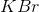. This molecule is made up of an alkali metal (potassium) and a halogen (bromine). Alkali metals have one valence electron that they readily lose to obtain octet whereas halogens have seven valence electrons and they readily gain an electron to obtain octet. Recall that losing an electron will give you aoxidation number whereas gaining an electron will give you aoxidation number. This means that alkali metals always have an oxidation number ofwhereas halogens always have an oxidation number of; therefore, potassium has an oxidation number of.

### Example Question #5 : Oxidation Reduction Reactions

Which of the following is the correct oxidation number of manganese in Manganese(III) oxide?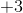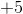Explanation:

Manganese(III) oxide has a formula of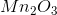. The oxidation number of oxygen is. Since there are three of them, the oxygen atoms contribute to a charge of. To maintain the neutrality of the molecule, manganese must balance thecharge (meaning contribute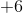charge). There are two manganese atoms; therefore, each manganese atom will have an oxidation number ofand contributecharge.

### Example Question #1 : Oxidation Reduction Reactions

A student reacts sodium chloride and lithium bromide. He collects the products in a jar and performs several tests on them. He concludes that product A has a metal and a nonmetal and that the metal has an oxidation number ofwhereas the nonmetal has an oxidation number of. What can you conclude from these results?

The other product will have similar oxidation numbers (metal:and nonmetal:)

The identity of product A is sodium bromide

The results seem invalid

The identity of product A is lithium chloride

The results seem invalid

Explanation:

The reaction stated in this question is as follows.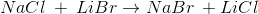This is a double replacement reaction. Both products contain a metal (an alkali metal) and a nonmetal (a halogen). The oxidation number of all alkali metals (first column of periodic table) isand of all halogens (seventh column of periodic table) is. The results stated in the question seem invalid because a halogen can never have an oxidation number of.

### Example Question #7 : Oxidation Reduction Reactions

In the compound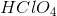, what is the oxidation number of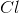?Generally, oxygen has an oxidation number of, and hydrogen has an oxidation number of. If we multiplyby the number of oxygen atoms in the compound (), we get an overall charge ofon the oxygen. If we multiplyby the number of hydrogen atoms in the compound (), we get an overall charge ofon the hydrogen. The sum ofandis. We can see thatis a neutral molecule, so the charge onwould have to balance out thecharge on the rest of the molecule. To obtain a charge of, the oxidation number onmust then be.